Saturday, August 15, 2020
Home > geometry > SSC CGL Geometry Shaded Area Set 3

# SSC CGL Geometry Shaded Area Set 3

SSC CGL CHSL Shared Area & Perimeter
Shaded Area & Perimeter Questions Sets
Set 1Set 2Set 3

SSC CGL Geometry Shaded Area Set 3 : Ques No 1

In the figure below, a square is of side 10 units, find the shaded area.Options:

A.

B.

C.

D.

Solution:

SSC CGL Geometry Shaded Area Set 3 : Ques No 2

In the figure below, two semi-circles are drawn in a square of side 20 units, find the shaded area.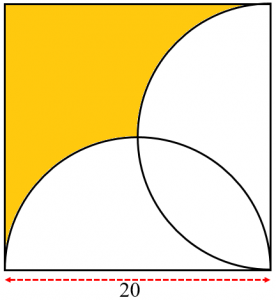Options:

A.

B.

C.

D.

Solution:

SSC CGL Geometry Shaded Area Set 3 : Ques No 3

In the figure below, a square is of side 4 units, find the shaded area.Options:

A.

B.

C.

D.

Solution:

SSC CGL Geometry Shaded Area Set 3 : Ques No 4

In the figure below, two semi-circles are drawn in a square of side 8 units, find the shaded area.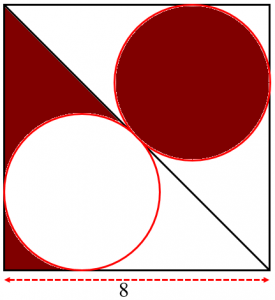Options:

A.

B.

C.

D.

Solution:

SSC CGL Geometry Shaded Area Set 3 : Ques No 5

In the figure below, a square is of side 4 units, find the shaded area.Options:

A.

B.

C.

D.

Solution:

SSC CGL Geometry Shaded Area Set 3 : Ques No 6

In the figure below, a square is of side 8 units, find the shaded area.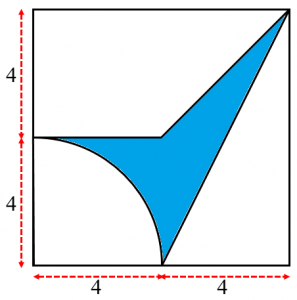Options:

A.

B.

C.

D.

Solution:SSC CGL Geometry Shaded Area Set 3 : Ques No 7

In the figure below, two semi-circles are drawn and CD is parallel to AB and CD = 32, find the shaded area.Options:

A.

B.

C.

D.

Solution:

SSC CGL Geometry Shaded Area Set 3 : Ques No 8

In the figure below, a rectangle PQRS is drawn in a circle. If PS = 8 units and PQ = 6 units, find the shaded area.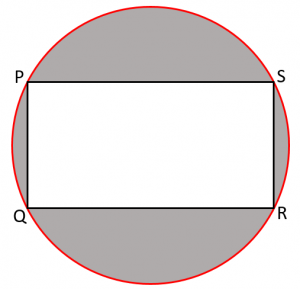Options:

A.

B.

C.

D.

Solution:

SSC CGL Geometry Shaded Area Set 3 : Ques No 9

In the figure below, two intersected circles passing through centers of each other, find the shaded area.Options:

A.

B.

C.

D.

Solution:

SSC CGL Geometry Shaded Area Set 3 : Ques No 10

In the figure below, find the shaded area.Options:

A.

B.

C.

D.

Solution:

SSC CGL Geometry Shaded Area Set 3 : Ques No 11

Figures I, II and III are squares. The perimeter of I is 12 and the perimeter of II is 24. The perimeter of III isOptions:

A. 9

B. 18

C. 36

D. 72

E. 81

SSC CGL Geometry Shaded Area Set 3 : Ques No 12

Triangles ABC, ADE, and EFG are all equilateral. Points D and G are midpoints of AC and AE,  respectively. If AB = 4, what is the perimeter of figure ABCDEFG?Options:

A. 12

B. 13

C. 15

D. 18

E. 21

SSC CGL Geometry Shaded Area Set 3 : Ques No 13

The perimeter of the polygon shown is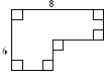Options:

A. 14

B. 20

C. 28

D. 48

E. cannot be determined from the information given

SSC CGL Geometry Shaded Area Set 3 : Ques No 14

The area of this figure is 100 cm2. (The figure consists of four identical squares.) Its perimeter is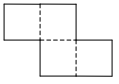Options:

A. 20

B. 25

C. 30

D. 40

E. 50

SSC CGL Geometry Shaded Area Set 3 : Ques No 15

What fraction of the square is shaded?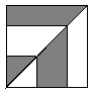Options:

A. 1/3

B. 2/5

C. 5/12

D. 3/7

E. 1/2

SSC CGL Geometry Shaded Area Set 3 : Ques No 16

Each of the three large squares shown below is the same size. Segments that intersect the sides of the squares intersect at the midpoints of the sides. How do the shaded areas of these squares compare?Options:

A. The shaded areas in all three are equal.

B. Only the shaded areas of I and II are equal.

C. Only the shaded areas of I and III are equal.

D. Only the shaded areas of II and III are equal.

E. The shaded areas of I, II and III are all different.